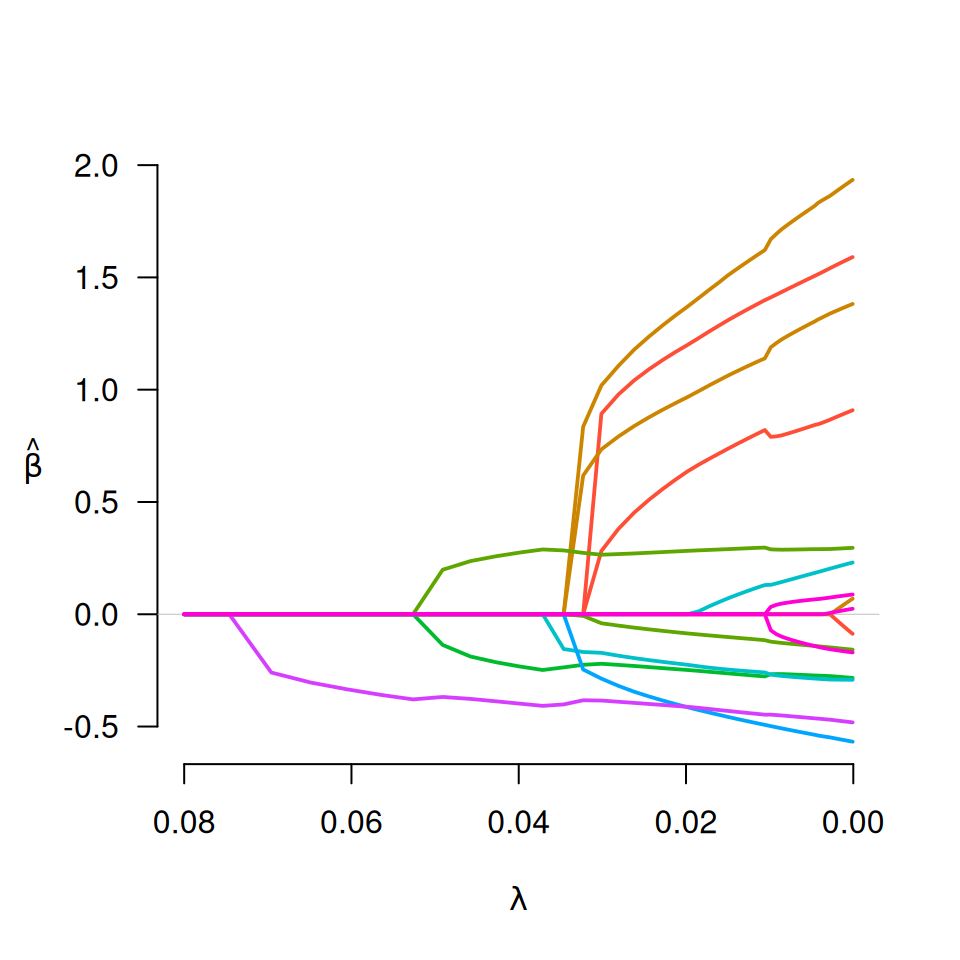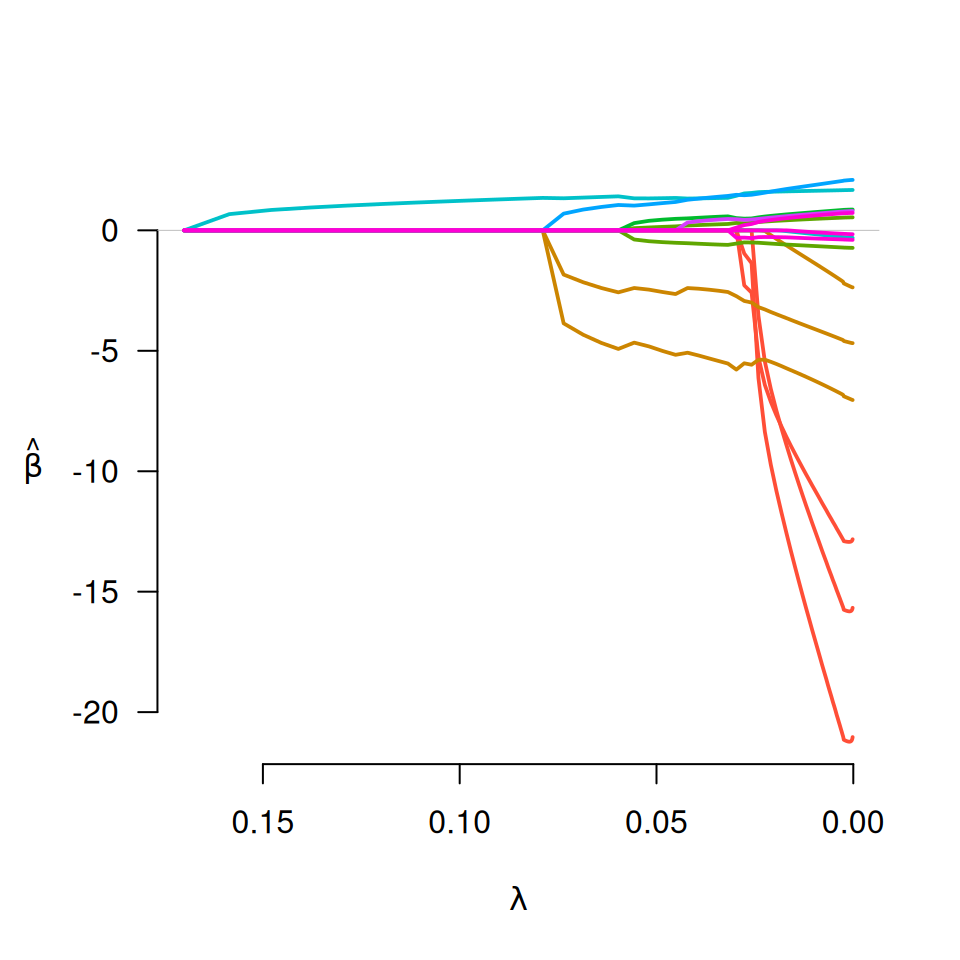Fit regularization paths for linear and logistic group bridge-penalized regression models over a grid of values for the regularization parameter lambda.

gBridge(X, y, group=1:ncol(X), family=c("gaussian", "binomial",
"poisson"), nlambda=100, lambda, lambda.min={if (nrow(X) > ncol(X)) .001
else .05}, lambda.max, alpha=1, eps=.001, delta=1e-7, max.iter=10000,
gamma=0.5, group.multiplier, warn=TRUE, returnX=FALSE, ...)

Arguments

X The design matrix, as in grpreg. The response vector (or matrix), as in grpreg. The grouping vector, as in grpreg. Either "gaussian" or "binomial", depending on the response. The number of lambda values, as in grpreg. A user supplied sequence of lambda values, as in grpreg. The smallest value for lambda, as in grpreg. The maximum value for lambda. Unlike the penalties in grpreg, it is not possible to solve for lambda.max directly with group bridge models. Thus, it must be specified by the user. If it is not specified, gBridge will attempt to guess lambda.max, but this is not particularly accurate. Tuning parameter for the balance between the group penalty and the L2 penalty, as in grpreg. Convergence threshhold, as in grpreg. The group bridge penalty is not differentiable at zero, and requires a small number delta to bound it away from zero. There is typically no need to change this value. Maximum number of iterations, as in grpreg. Tuning parameter of the group bridge penalty (the exponent to which the L1 norm of the coefficients in the group are raised). Default is 0.5, the square root. The multiplicative factor by which each group's penalty is to be multiplied, as in grpreg. Should the function give a warning if it fails to converge? As in grpreg. Return the standardized design matrix (and associated group structure information)? Default is FALSE. Not used.

Details

This method fits the group bridge method of Huang et al. (2009). Unlike the penalties in grpreg, the group bridge is not differentiable at zero; because of this, a number of changes must be made to the algorithm, which is why it has its own function. Most notably, the method is unable to start at lambda.max; it must start at lambda.min and proceed in the opposite direction.

In other respects, the usage and behavior of the function is similar to the rest of the grpreg package.

Value

An object with S3 class "grpreg", as in grpreg.

• Huang J, Ma S, Xie H, and Zhang C. (2009) A group bridge approach for variable selection. Biometrika, 96: 339-355. doi: 10.1093/biomet/asp020

• Breheny P and Huang J. (2009) Penalized methods for bi-level variable selection. Statistics and its interface, 2: 369-380. doi: 10.4310/sii.2009.v2.n3.a10

Author

Patrick Breheny

data(Birthwt) X <- Birthwt$X group <- Birthwt$group ## Linear regression y <- Birthwt$bwt fit <- gBridge(X, y, group) plot(fit)select(fit)$beta
## Logistic regression y <- Birthwt$low fit <- gBridge(X, y, group, family="binomial") plot(fit)select(fit)$beta